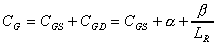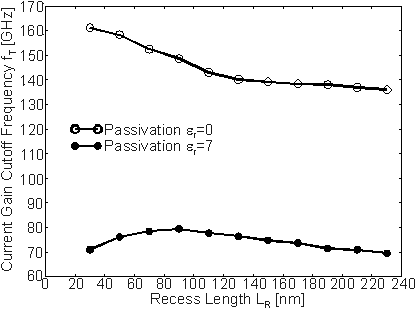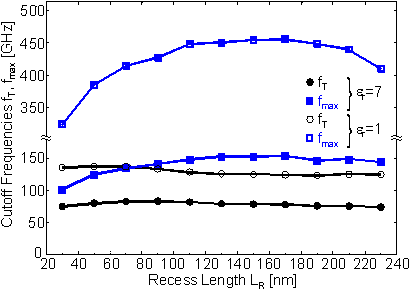Next: 6.2 Power HEMT for 0.9/1.9 GHz and 40 GHz Applications Up: 6.1.2 RF Characteristics Previous: 6.1.2.2 Contributions to the Gate Capacitance

6.1.2.3 Reduction of the Recess Length

As demonstrated in Section 6.1.1, gm  ext is increased when LR is reduced. The reason is a decrease of the ohmic resistance in the current path. Therefore, gm is expected to depend roughly linearly on LR. It is found that the dependence of CG on LR can be modeled by(63)
where a and b are constants. The simulated dependence of fT on LR is plotted in  Figure 6.17. In the hypothetical situation er = 0, there is almost no dependence of CG on LR, and fT increases monotonously with decreasing LR due to the improvement of gm ext. For the case that the gate and the adjacent device surfaces are encapsulated by a dielectric with er = 7, the decrease of fT on the left hand side of the maximum at LR  90 nm is caused by the rapidly increasing CGD. If a device passivation with a dielectric constant er < 7 were available, it is evident that fT would be higher for any er < 7 and the optimum of LR would be shifted towards smaller values.Figure 6.17 Simulated fT versus LR for a passivated HEMT with er = 7 (filled symbols) and er = 0 (open symbols) at VGS = 0.2 V and VDS = 2.0 V

The rapid increase in CGD has even a more significant impact on fmax. To determine fmax RS is calculated by the assumption that the intrinsic gmi remains constant and the reduction of the extrinsic gm is purely attributed to an increase of RS with LR. The gate resistance is assumed to be independent of LR and is taken to be zero in the simulations. To calculate fmax the measured value of RG of HEMT A is taken. In  Figure 6.18 fT and fmax are shown as a function of LR for fully passivated (er = 7) and unpassivated devices (er = 1). For er = 7 and small LR CGD is very large due to the small distance between the gate and drain contact metals which leads to rather low fT and fmax. Both fT and fmax increases with increasing LR and reach a local maximum. But the maximum of fmax at LR = 150 nm is obtained for significant larger LR than the maximum for fT. The reason is that fmax depends much stronger on CGD than fT. Therefore, a decrease of CGD is overcompensated by the linear increase in RS for larger values of LR.Figure 6.18 Simulated fT and fmax versus LR for a passivated HEMT with er = 7 (circles) and er = 1 (squares) at VGS = 0.2 V and VDS = 2.0 V

The investigations on low noise HEMTs show very good agreement between the calculated and measured characteristics of the devices. It is possible to trace differences in manufactured devices, for instance, the recess depth with an accuracy in the order of 1 nm. Therefore, very accurate information for improvements of device characteristics or the technology of these devices can be given.

All devices were based on a homogeneously doped single heterojunction structure, i. e. a GaAs/InGaAs/AlGaAs layer sequence. As already discussed the disadvantage of this sequence is that the energy barrier on the backside of the channel is rather low which limits the power capability. In the following sections HEMTs based on delta doped double heterojunction structures similar to the structure of HEMTref will be investigated.Next: 6.2 Power HEMT for 0.9/1.9 GHz and 40 GHz Applications Up: 6.1.2 RF Characteristics Previous: 6.1.2.2 Contributions to the Gate Capacitance

Helmut Brech
1998-03-11